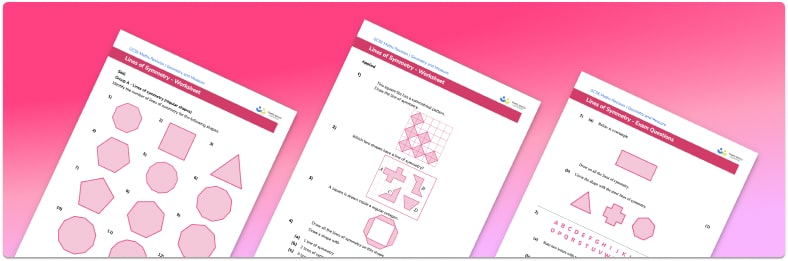# Lines of Symmetry Worksheet• Section 1 of the lines of symmetry worksheet contains 27 skills-based lines of symmetry questions, in 3 groups to support differentiation
• Section 2 contains 4 applied lines of symmetry questions with a mix of worded symmetry activities and deeper problem solving questions
• Section 3 contains 3 foundation and higher level GCSE exam style lines of symmetry questions
• Answers and a mark scheme for all lines of symmetry questions are provided
• Questions follow variation theory with plenty of opportunities for students to work independently at their own level
• All questions created by fully qualified expert secondary maths teachers
• Suitable for GCSE maths revision for AQA, OCR and Edexcel exam boards

• This field is for validation purposes and should be left unchanged.

You can unsubscribe at any time (each email we send will contain an easy way to unsubscribe). To find out more about how we use your data, see our privacy policy.

### Lines of symmetry at a glance

A shape has a line of symmetry if a line can be drawn through the shape so that each half of the shape is an exact mirror image of the other half (identical halves). Some 2D shapes have multiple lines of symmetry, some have one and others don’t have any.

We might be asked to draw lines of symmetry on different shapes or to identify the number of lines of symmetry a polygon may have. We could also be asked to create symmetrical shapes by adding sections or shading parts of a diagram.

Regular polygons have a number of lines of symmetry equal to their number of sides. For example, an equilateral triangle has three lines of symmetry and a regular hexagon has six lines of symmetry. Drawing a symmetric figure in different orientations will not change its lines of symmetry.

Usually diagrams are placed on grids or a set of xy axes.

Vertical lines of symmetry can be labelled as x=a where a is an integer or a decimal.

Horizontal lines of symmetry can be labelled as y=b where b is an integer or a decimal.

Two types of shape symmetry include line symmetry and rotational symmetry.

Looking forward, students can then progress to additional symmetry worksheets and other geometry worksheets, for example a simplifying expressions worksheet or simultaneous equations worksheet.For more teaching and learning support on Geometry our GCSE maths lessons provide step by step support for all GCSE maths concepts.

## Do you have KS4 students who need more focused attention to succeed at GCSE?There will be students in your class who require individual attention to help them succeed in their maths GCSEs. In a class of 30, it’s not always easy to provide.

Help your students feel confident with exam-style questions and the strategies they’ll need to answer them correctly with our dedicated GCSE maths revision programme.

Lessons are selected to provide support where each student needs it most, and specially-trained GCSE maths tutors adapt the pitch and pace of each lesson. This ensures a personalised revision programme that raises grades and boosts confidence.

Find out more